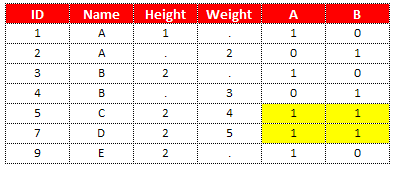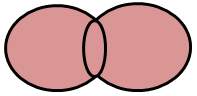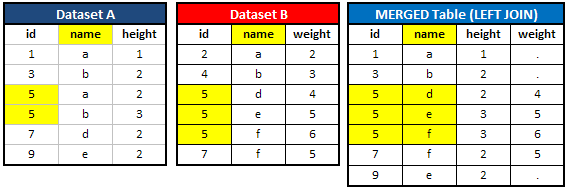# SAS Merging Tutorial (With Examples)

This tutorial explains how to merge datasets in SAS. It explains the different types of joins with MERGE statement. Also it includes some special topics related to merging.

Introduction: Data Step Merge

Data step merge is used to merge two or more datasets based on one or more common variables. It is performed using the MERGE statement within DATA step in SAS. It is similar to SQL joins.

Create 2 Sample Datasets

The following SAS code is used to create two sample SAS datasets that will be used to demonstrate merging in SAS.

Data A;
Input ID Name\$ Height;
cards;
1 A 1
3 B 2
5 C 2
7 D 2
9 E 2
;
run;
Data B;
Input ID Name\$ Weight;
cards;
2 A 2
4 B 3
5 C 4
7 D 5
;
run;

## Important Steps when using MERGE Statement in SAS

1. Step 1 : Both the data sets must be SORTED by the variable you want to use for merging.
2. Step 2 : The variable you want to use for merging must have same name in both the datasets.

Let's merge dataset A and B

First, Sort both the datasets with PROC SORT. See the code below -

proc sort data = a;
by id;
run;
proc sort data = b;
by id;
run;

Next Step : Use MERGE statement to merge the datasets by the variable ID.

data dummy;
merge a (in=x) b(in=y);
by id;
a = x;
b = y;
run;
What is IN= option in Data Step Merge?

The IN= option tells SAS to create a flag that has either the value 0 or 1. If the observation does not come from the dataset, then the flag returns 0. If the observation comes from the data set, then the flag returns 1.

Since the IN= option creates temporary variables, we need to create permanent variables so that we can see the flag in the dataset. With this lines of code "a = x; b = y;", we tell SAS to create two variables named a, b and put the same values as stored in variables x and y. You can assign any name you want, not just a.b. See the Output shown in the image below -Output

In the above image, the highlighted yellow rows are the rows that are common in both the datasets. Hence, the values are 1 in variables A and B. The value 1 in variable A implies these rows come from dataset A and 0 implies these rows do not come from dataset A. The same logic holds for variable B. When variable B has 1, it means these rows come from dataset B.

What Happens If You Don't Include 'BY' Statement in Merging Data?

The BY Statement tells SAS to match records based on the common variable you specify. Without the 'BY' statement, it does not perform matching of records. What would happen? Observations are combined based on their relative position in each data set. For example, observation one from the first data set combines with observation one of the second data set, the second observation from the first data set combines with the second observation from the second data set, and so on.MERGE without BY Statement

If there is a common variable in the two datasets, the value is overwritten by the value in the right dataset. Since ID and Name are the common variables, the values are overwritten by dataset B.

The number of observations in the combined dataset is equal to the number of observations in the dataset with largest number of observations. For example, dataset A has 5 observations and dataset B has 4 observations so final data would have 5 observations.

Types of JOINS

In this section, we cover different types of joins using the MERGE statement in SAS.

## SAS : INNER JOIN

Inner Join : It returns rows common to both tables (data sets). In the final merged file, number of columns would be (Common columns in both the data sets + uncommon columns from data set A + uncommon columns from data set B).INNER JOIN

proc sort data = a;
by id;
run;

proc sort data = b;
by id;
run;

Data dummy;
Merge A (IN = X) B (IN=Y);
by ID;
If X and Y;
run;

Note : When using IN= option, SAS considers "If X and Y" equivalent to "If X=1 and Y=1".Data Step Merge : INNER JOIN Example

Explanation

Since the above case is of INNER JOIN, Data Step Merge returns values 5 and 7 which are common in variable ID of both the datasets.

## SAS : LEFT JOIN

Left Join : It returns all rows from the left table and the matched rows from the right table.LEFT JOIN
proc sort data = a;
by id;
run;

proc sort data = b;
by id;
run;

Data dummy;
Merge A (IN = X) B (IN=Y);
by ID;
If X ;
run;

Note : When you use IN= option, SAS considers "If X" equivalent to "If X=1". We can use either of the If statement.Data Step Merge : LEFT JOIN Example
Explanation

Since the above case is of LEFT JOIN, Data Step Merge returns all observations from dataset A with matching rows from dataset B.

## SAS : RIGHT JOIN

Right Join : It returns all rows from the right table, and the matched rows from the left table.RIGHT JOIN
proc sort data = a;
by id;
run;

proc sort data = b;
by id;
run;

Data dummy;
Merge A (IN = X) B (IN=Y);
by ID;
If Y ;
run;

Explanation

Since the above case is of RIGHT JOIN, Data Step Merge returns all observations from dataset B with matching rows from dataset A.

## SAS : FULL JOIN

Full Join: It returns all rows from the left table and from the right table.FULL JOIN
proc sort data = a;
by id;
run;

proc sort data = b;
by id;
run;

Data dummy;
Merge A B;
by ID;
run;

Note : Since the FULL JOIN is the default type of JOIN in MERGE Statement, it does not require temporary variables with IN option.

Explanation

Since the above case is of FULL JOIN, Data Step Merge returns all observations from dataset A and B.

What happens when the BY variable in Data Step Merge has different length?

When we merge datasets with BY variable having different lengths, the length of the BY variable used during matching is determined by the left-hand side dataset in the merge. If length of dataset A is shorter than B, it may return zero records.

Solution - Include bigger length of the common variable with LENGTH Statement before MERGE statement.

data dummy;
length ID 8;
merge a b;
by id;
run;
Special Cases
1. If both the tables (data sets) have similar variable name (other than primary key), Data Step MERGE statement would take values of the common variable exist in the TABLE2 (Right table).
2. If primary key in both the tables (data sets) have duplicate values, Data Step MERGE statement would return a maximum number of values in both the tables. For example, Table 1 has 3 1's and Table 2 has 2 1's, Data Step Merge would return 3 1's. It is called 'One-to-Many Merge'.
See the special case shown in the image below -Special Cases : Data Step Merge

In this case, dataset A contains two 5s and dataset B contains three 5s. When we merged these two tables, it returns three 5s which is maximum number of 5s in both the dataset A and B.

Important Point - Did you notice the variable "name" exists in both the datasets A and B? In this example, the variable "name" is NOT a primary key to merge the tables. It is the variable "id" which is the primary key to merge these tables. When we merged the tables, DATA STEP MERGE takes values of variable "name" from dataset B.

SAS Code for the above special case
data a;
input id name\$ height;
cards;
1 a 1
3 b 2
5 a 2
5 b 3
7 d 2
9 e 2
;
run;
data b;
input id name\$ weight;
cards;
2 a 2
4 b 3
5 d 4
5 e 5
5 f 6
7 f 5
;
run;

data c;
merge a (in=x) b(in=y);
by id;
if x;
proc print;
run;
Q. Do the "Special Cases" explained above hold true for all types of joins?

Answer is YES. It holds true for all the types of joins.

How to check if merge was done correctly?
1. Before merging, ask yourself whether the variable type and length of the BY variable is same.
2. First check the number of observations in the input files and estimate the number of observations should come in the final merged data set.
3. Check the number of variables in the input files and estimate the number of variables should appear in the final merged data set.
4. If there are duplicates in the BY variable of the input files, how data step merge has considered these cases? Whether you are getting the desired output?

Check out : Merging with Proc SQL

Related Posts
ShareDeepanshu founded ListenData with a simple objective - Make analytics easy to understand and follow. He has over 10 years of experience in data science. During his tenure, he worked with global clients in various domains like Banking, Insurance, Private Equity, Telecom and HR.

21 Responses to "SAS Merging Tutorial (With Examples)"
1.1.2.Great Be more descriptive

3.4.kindly check important notes 1 & 2 are same for all examples.

1.It is because it holds true for all the types of joins. I have added more explanation with example to make it easy. Hope it helps!

5.thanks ! great article

6.Thanks Deepanshu, the content was really descriptive and very helpful.

7.in the above example,
when merging two ds , how come the third row got 3 as height???
after doing row-by-row matching, third row (5 f 6) of ds2 will not get any value for height in ds1 ? then how come it is getting 3?

ds1
id name height
5 a 2
5 b 3

ds2
id name weight
5 d 4
5 e 5
5 f 6

ds3
id name height weight
5 d 2 4
5 e 3 5
5 f 3 6

id name height weight
5 d 2 4
5 e 3 5
5 f . 6

1.In this case, dataset A contains two 5s and dataset B contains three 5s. When we merged these two tables, it returns three 5s which is maximum number of 5s in both the dataset A and B.

in this data values of same variable will be overwritten by second dataset in first dataset.

8.9.10.Only started learning SAS a few days ago and these pages are the best! Thanks heaps for all of your efforts to make them :)

11.Thanks for ur example and show todoy . i start sas code and understand.

12.Hi Deepanshu,
Wnated your help in creating a dashboard with linking such that I click on one option and it takes me to the hyperlinked database.

How to do this

13.14.very good explanation.really useful.

15.Thanks for this! It was really helpful!

16.when we specify if y, it means right join. But What kind of join will it be if I mention 'if not y'?

17.hi , i am still confused with the special cases. you mentioned that in special cases, if the datasets have common variables apart from the variable used for merging, isn't this the case in the previous examples where we had id name height in a dataset and id name weight in the other dataset, there we did not take variables of name from b dataset in case of merging.

18.In the special cases example why are we subsetting by x? shouldnt it be y given the conditions mentioned under special cases?

Next → ← Prev